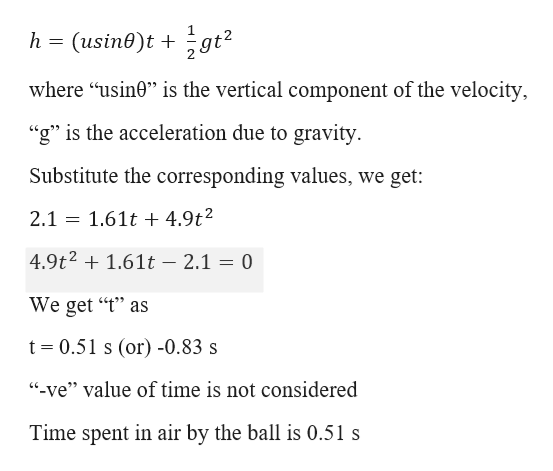# A boy throws a baseball onto a roof and it rolls back down and off the roof with a speed of 4.30 m/s. If the roof is pitched at 22.0° below the horizon and the roof edge is 2.10 m above the ground, find the time the baseball spends in the air and the horizontal distance from the roof edge to the point where the baseball lands on the ground.

Question
47 views

A boy throws a baseball onto a roof and it rolls back down and off the roof with a speed of 4.30 m/s. If the roof is pitched at 22.0° below the horizon and the roof edge is 2.10 m above the ground, find the time the baseball spends in the air and the horizontal distance from the roof edge to the point where the baseball lands on the ground.

check_circle

Step 1

Given information:

Velocity of the ball before leaving the roof (u) = 4.30 m/s

Angle of the roof (θ) = 22.00

Height of the roof from the ground (h) = 2.10 m

Step 2

Time the ball spent in are:

Consider 2nd...help_outlineImage Transcriptioncloseh (usin0)t 9t2 where "usin0" is the vertical component of the velocity, "g" is the acceleration due to gravity Substitute the corresponding values, we get: 1.61t4.9t2 2.1 4.9t21.61t - 2.1 = 0 We get "t" as t 0.51 s (or) -0.83 s ve" value of time is not considered Time spent in air by the ball is 0.51 s fullscreen

### Want to see the full answer?

See Solution

#### Want to see this answer and more?

Solutions are written by subject experts who are available 24/7. Questions are typically answered within 1 hour.*

See Solution
*Response times may vary by subject and question.
Tagged in

### Kinematics